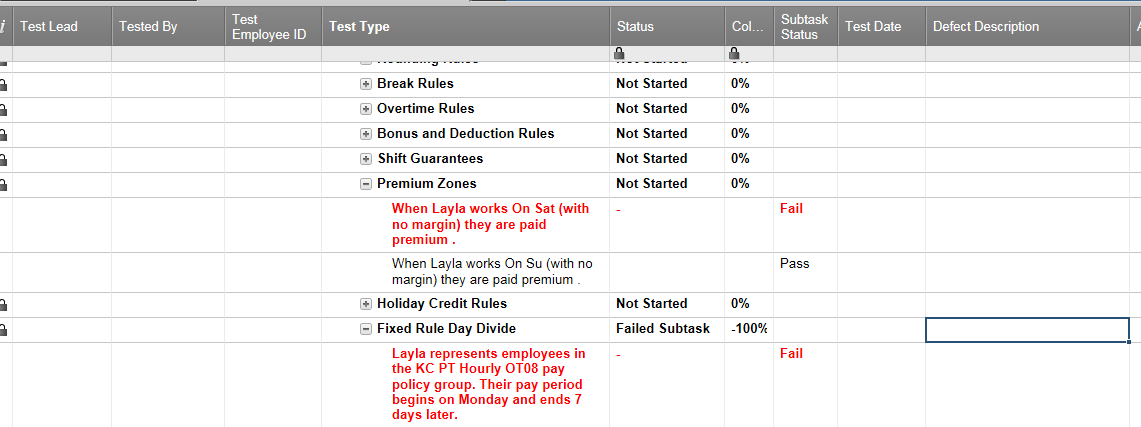#### Welcome to the Smartsheet Forum Archives

The posts in this forum are no longer monitored for accuracy and their content may no longer be current. If there's a discussion here that interests you and you'd like to find (or create) a more current version, please Visit the Current Forums.

# IF Match formula failing. Can you use Children as the test to search?

=IF(FIND("Fail", (CHILDREN([Subtask Status]231))) > 0, "Failed Subtask", IF(Column231 = 1, "Pass", IF(Column231 = 0, "Not Started", IF(Column231 > 0, "Review In Progress", IF(Column231 < 0, "Review in Progress")))))

The above formula is returning #Invalid Data Type

I also tried to break the formula down into two parts to test each part of =IF(FIND("Fail", (CHILDREN([Subtask Status]231))) > 0, "Failed Subtask", "No") results is #Invalid Data type

=IF(Column231 = 1, "Pass", IF(Column231 = 0, "Not Started", IF(Column231 > 0, "Review In Progress", IF(Column231 < 0, "Failed Subtask")))) results are correct

Desired outcome

IF "Fail" is in the children of Subtask Status231 return "Failed Subtask" otherwise IF Column231=0 return Not started, IF Column231>0 return In Progress, IF column231<0 return Failed Subtask

Screen grab shows the two scenarios and the reason I need to add the Find function to the calculation• This is basically the same as your previous question.  It looks like you've dropped the "Pass" checkbox column, but you can still get the results you want using the following.

For the "Column" formula, use: